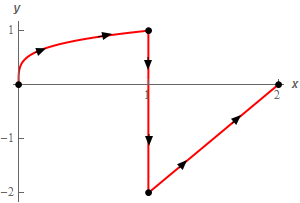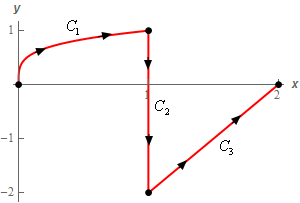Paul's Online Notes
Home / Calculus III / Line Integrals / Line Integrals - Part I
Show Mobile Notice Show All Notes Hide All Notes
Mobile Notice
You appear to be on a device with a "narrow" screen width (i.e. you are probably on a mobile phone). Due to the nature of the mathematics on this site it is best views in landscape mode. If your device is not in landscape mode many of the equations will run off the side of your device (should be able to scroll to see them) and some of the menu items will be cut off due to the narrow screen width.

### Section 16.2 : Line Integrals - Part I

6. Evaluate $$\displaystyle \int\limits_{C}{{16{y^5}\,ds}}$$ where $$C$$ is the portion of $$x = {y^4}$$ from $$y = 0$$ to $$y = 1$$ followed by the line segment form $$\left( {1,1} \right)$$ to $$\left( {1, - 2} \right)$$ which in turn is followed by the line segment from $$\left( {1, - 2} \right)$$ to $$\left( {2,0} \right)$$. See the sketch below for the direction.Show All Steps Hide All Steps

Start Solution

To help with the problem let’s label each of the curves as follows,Now let’s parameterize each of these curves.

$${C_1}:\,\,\vec r\left( t \right) = \left\langle {{t^4},t} \right\rangle \hspace{0.25in}0 \le t \le 1$$
$${C_2}:\,\,\vec r\left( t \right) = \left( {1 - t} \right)\left\langle {1,1} \right\rangle + t\left\langle {1, - 2} \right\rangle = \left\langle {1,1 - 3t} \right\rangle \hspace{0.25in}0 \le t \le 1$$
$${C_3}:\,\,\vec r\left( t \right) = \left( {1 - t} \right)\left\langle {1, - 2} \right\rangle + t\left\langle {2,0} \right\rangle = \left\langle {1 + t,2t - 2} \right\rangle \hspace{0.25in}0 \le t \le 1$$

For $${C_2}$$ we had to use the vector form for the line segment between two points instead of the equation for the line (which is much simpler of course) because the direction was in the decreasing $$y$$ direction and the limits on our integral must be from smaller to larger. We could have used the fact from the notes that tells us how the line integrals for the two directions related to allow us to use the equation of the line if we’d wanted to. We decided to do it this way just for the practice of dealing with the vector form for the line segment and it’s not all that difficult to deal with the result and the limits are “nicer”.

Note as well that for $${C_3}$$ we could have solved for the equation of the line and used that because the direction is in the increasing $$x$$ direction. However, the vector form for the line segment between two points is just as easy to use so we used that instead.

Show Step 2

Okay, we now need to compute the line integral along each of these curves. Unlike the first few problems in this section where we found the magnitude and the integrand prior to the integration step we’re just going to just straight into the integral and do all the work there.

Here is the integral along each of the curves.

\begin{align*}\int\limits_{{{C_1}}}{{16{y^5}\,ds}} & = \int_{0}^{1}{{16{{\left( t \right)}^5}\,\sqrt {{{\left( {4{t^3}} \right)}^2} + {{\left( 1 \right)}^2}} \,dt}} = \int_{0}^{1}{{16{t^5}\,\sqrt {16{t^6} + 1} \,dt}}\\ & = \left. {\frac{1}{9}{{\left( {16{t^6} + 1} \right)}^{\frac{3}{2}}}} \right|_0^1 = \underline {\frac{1}{9}\left( {{{17}^{\frac{3}{2}}} - 1} \right) = 7.6770} \end{align*} \begin{align*}\int\limits_{{{C_2}}}{{16{y^5}\,ds}} &= \int_{0}^{1}{{16{{\left( {1 - 3t} \right)}^5}\,\sqrt {{{\left( 0 \right)}^2} + {{\left( { - 3} \right)}^2}} \,dt}} = \int_{0}^{1}{{48{{\left( {1 - 3t} \right)}^5}\,\,dt}}\\ & = \left. { - \frac{8}{3}{{\left( {1 - 3t} \right)}^6}} \right|_0^1 = \underline { - 168} \end{align*} \begin{align*}\int\limits_{{{C_3}}}{{16{y^5}\,ds}} &= \int_{0}^{1}{{16{{\left( {2t - 2} \right)}^5}\,\sqrt {{{\left( 1 \right)}^2} + {{\left( 2 \right)}^2}} \,dt}} = \int_{0}^{1}{{16\sqrt 5 {{\left( {2t - 2} \right)}^5}\,\,dt}}\\ & = \left. {\frac{{4\sqrt 5 }}{3}{{\left( {2t - 2} \right)}^6}} \right|_0^1 = \underline { - \frac{{256\sqrt 5 }}{3} = - 190.8111} \end{align*} Show Step 3

Okay to finish this problem out all we need to do is add up the line integrals over these curves to get the full line integral.

$\int\limits_{C}{{16{y^5}\,ds}} = \left( {\frac{1}{9}\left( {{{17}^{\frac{3}{2}}} - 1} \right)} \right) + \left( { - 168} \right) + \left( { - \frac{{256\sqrt 5 }}{3}} \right) = \require{bbox} \bbox[2pt,border:1px solid black]{{ - 351.1341}}$

Note that we put parenthesis around the result of each individual line integral simply to illustrate where it came from and they aren’t needed in general of course.